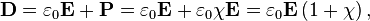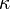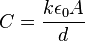# Dielectric

A dielectric, or electrical insulator, is a material that is highly resistant to the flow of an electric current. Dielectric materials can be solids, liquids, or gases. In addition, a vacuum is an excellent dielectric.

An important application of dielectrics is to separate the plates of capacitors. A capacitor's ability to store electric charge depends on the dielectric that separates its plates.

## Explanation

When a dielectric medium interacts with an applied electric field, charges are redistributed within its atoms or molecules. This redistribution alters the shape of an applied electrical field both within the dielectric medium and in the nearby region.

When two electric charges move through a dielectric medium, the interaction energies and forces between them are reduced. When an electromagnetic wave travels through a dielectric, its speed decreases and its wavelength shortens.

When an electric field is initially applied across a dielectric medium, a current flows. The total current flowing through a real dielectric is made up of two parts: a conduction and a displacement current. In good dielectrics, the conduction current will be extremely small. The displacement current can be considered the elastic response of the dielectric material to any change in the applied electric field. As the magnitude of the electric field is increased, a displacement current flows, and the additional displacement is stored as potential energy within the dielectric. When the electric field is decreased, the dielectric releases some of the stored energy as a displacement current. The electric displacement can be separated into a vacuum contribution and one arising from the dielectric by$\mathbf{D} = \varepsilon_{0} \mathbf{E} + \mathbf{P} = \varepsilon_{0} \mathbf{E} + \varepsilon_{0}\chi\mathbf{E} = \varepsilon_{0} \mathbf{E} \left( 1 + \chi \right),$

where P is the polarization of the medium, E is the electric field, D is the electric flux density (or displacement), and$\chi$ its electric susceptibility. It follows that the relative permittivity and susceptibility of a dielectric are related,$\varepsilon_{r} = \chi + 1$.

Material Dielectric constant
Vacuum 1 (by definition)
Air 1.00054
Teflon™ 2.1
Polyethylene 2.25
Polystyrene 2.4–2.7
Paper 3.5
Concrete 4.5
Pyrex (glass) 4.7 (3.7–10)
Rubber 7
Diamond 5.5–10
Salt 3–15
Graphite 10–15
Silicon 11.68
Methanol 30
Furfural 42.0
Glycerol 47–68
Water 88–80.1–55.3–34.5
(0–20–100–200 °C)
Hydrofluoric acid 83.6 (0 °C)
Formamide 84.0 (20 °C)
Sulfuric acid 84–100
(20–25 °C)
Hydrogen peroxide 128 aq–60
(−30–25 °C)
Hydrocyanic acid 158.0–2.3
(0–21 °C)
Titanium dioxide 86–173
Strontium titanate 310
Barium strontium titanate 15 nc–500
Barium titanate 90 nc–1250–10,000
(20–120 °C)
(La,Nb):(Zr,Ti)PbO3 500,6000

### Dielectric constant

The dielectric constant (or static permittivity) of a material (under given conditions) is a measure of the extent to which the material concentrates electrostatic lines of flux. In practice, it is measured as the "relative dielectric constant," which is defined as the ratio of the amount of electrical energy stored in an insulator when a static electric field is imposed across it, relative to the permittivity of a vacuum (which has a dielectric constant of 1).

The relative dielectric constant is represented as εr (or sometimes$\kappa$, K, or Dk). Mathematicallly, it is defined as:$\varepsilon_{r} = \frac{\varepsilon_{s}}{\varepsilon_{0}}$

where εs is the static permittivity of the material, and ε0 is vacuum permittivity. Vacuum permittivity is derived from Maxwell's equations by relating the electric field intensity E to the electric flux density D. In vacuum (free space), the permittivity ε is just ε0, so the dielectric constant is one.

### Permittivity

Permittivity is a physical quantity that describes how an electric field affects and is affected by a dielectric medium, and is determined by the ability of a material to polarize in response to the field, and thereby reduce the field inside the material. Thus, permittivity relates to a material's ability to transmit (or "permit") an electric field.

It is directly related to electric susceptibility. For example, in a capacitor, an increased permittivity allows the same charge to be stored with a smaller electric field (and thus a smaller voltage), leading to an increased capacitance.

## Dielectric strength

The term dielectric strength may be defined as follows:

• For an insulating material, dielectric strength is the maximum electric field strength that the material can withstand intrinsically without breaking down, that is, without experiencing failure of its insulating properties.
• For a given configuration of dielectric material and electrodes, dielectric strength is the minimum electric field that produces breakdown.

The theoretical dielectric strength of a material is an intrinsic property of the bulk material and is dependent on the configuration of the material or the electrodes with which the field is applied. At breakdown, the electric field frees bound electrons. If the applied electric field is sufficiently high, free electrons may become accelerated to velocities that can liberate additional electrons during collisions with neutral atoms or molecules in a process called avalanche breakdown. Breakdown occurs quite abruptly (typically in nanoseconds), resulting in the formation of an electrically conductive path and a disruptive discharge through the material. For solid materials, a breakdown event severely degrades, or even destroys, its insulating capability.

### Breakdown field strength

The field strength at which breakdown occurs in a given case is dependent on the respective geometries of the dielectric (insulator) and the electrodes with which the electric field is applied, as well as the rate of increase at which the electric field is applied. Because dielectric materials usually contain minute defects, the practical dielectric strength will be a fraction of the intrinsic dielectric strength seen for ideal, defect free, material. Dielectric films tend to exhibit greater dielectric strength than thicker samples of the same material. For instance, dielectric strength of silicon dioxide films of a few hundred nm to a few microns thick is approximately ten MV/cm. Multiple layers of thin dielectric films are used where maximum practical dielectric strength is required, such as high voltage capacitors and pulse transformers.

Dielectric strength of various common materials
Material Dielectric Strength (MV/m)
Air 3
Quartz 8
Strontium titanate 8
Neoprene rubber 12
Nylon 14
Pyrex glass 14
Silicone oil 15
Paper 16
Bakelite 24
Polystyrene 24
Teflon 60

## Dielectrics in Parallel-Plate CapacitorsThe electrons in the molecules shift toward the positively charged left plate. The molecules then create a leftward electric field that partially cancels the field created by the plates. (The air gap is shown for clarity; in real capacitor, the dielectric is usually in direct contact with the plates.)

Putting a dielectric material between the plates in a parallel plate capacitor causes an increase in the capacitance in proportion to k, the relative permittivity of the material:$C = \frac{k \epsilon_0 A}{d}$
where$\epsilon_0$ is the permittivity of free space, A is the area covered by the capacitors, and d is the distance between the plates.

This happens because an electric field polarizes the bound charges of the dielectric, producing concentrations of charge on its surfaces that create an electric field opposed (antiparallel) to that of the capacitor. Thus, a given amount of charge produces a weaker electric field between the plates than it would without the dielectric, which reduces the electric potential. Considered in reverse, this argument means that, with a dielectric, a given electric potential causes the capacitor to accumulate a larger charge polarization.

## Applications

The use of a dielectric in a capacitor presents several advantages. The simplest of these is that the conducting plates can be placed very close to one another without risk of contact. Also, if subjected to a very high electric field, any substance will ionize and become a conductor. Dielectrics are more resistant to ionization than dry air, so a capacitor containing a dielectric can be subjected to a higher operating voltage. Layers of dielectric are commonly incorporated in manufactured capacitors to provide higher capacitance in a smaller space than capacitors using only air or a vacuum between their plates, and the term dielectric refers to this application as well as the insulation used in power and RF cables.

## Some practical dielectrics

Dielectric materials can be solids, liquids, or gases. In addition, a high vacuum can also be a useful, lossless dielectric even though its relative dielectric constant is only unity.

Solid dielectrics are perhaps the most commonly used dielectrics in electrical engineering, and many solids are very good insulators. Some examples include porcelain, glass, and most plastics. Air, nitrogen and sulfur hexafluoride are the three most commonly used gaseous dielectrics.

• Industrial coatings such as parylene provide a dielectric barrier between the substrate and its environment.
• Mineral oil is used extensively inside electrical transformers as a fluid dielectric and to assist in cooling. Dielectric fluids with higher dielectric constants, such as electrical grade castor oil, are often used in high voltage capacitors to help prevent corona discharge and increase capacitance.
• Because dielectrics resist the flow of electricity, the surface of a dielectric may retain stranded excess electrical charges. This may occur accidentally when the dielectric is rubbed (the triboelectric effect). This can be useful, as in a Van de Graaff generator or electrophorus, or it can be potentially destructive as in the case of electrostatic discharge.
• Specially processed dielectrics, called electrets, may retain excess internal charge or "frozen in" polarization. Electrets have a semipermanent external electric field, and are the electrostatic equivalent to magnets. Electrets have numerous practical applications in the home and industry.
• Some dielectrics can generate a potential difference when subjected to mechanical stress, or change physical shape if an external voltage is applied across the material. This property is called piezoelectricity. Piezoelectric materials are another class of very useful dielectrics.
• Some ionic crystals and polymer dielectrics exhibit a spontaneous dipole moment which can be reversed by an externally applied electric field. This behavior is called the ferroelectric effect. These materials are analogous to the way ferromagnetic materials behave within an externally applied magnetic field. Ferroelectric materials often have very high dielectric constants, making them quite useful for capacitors.